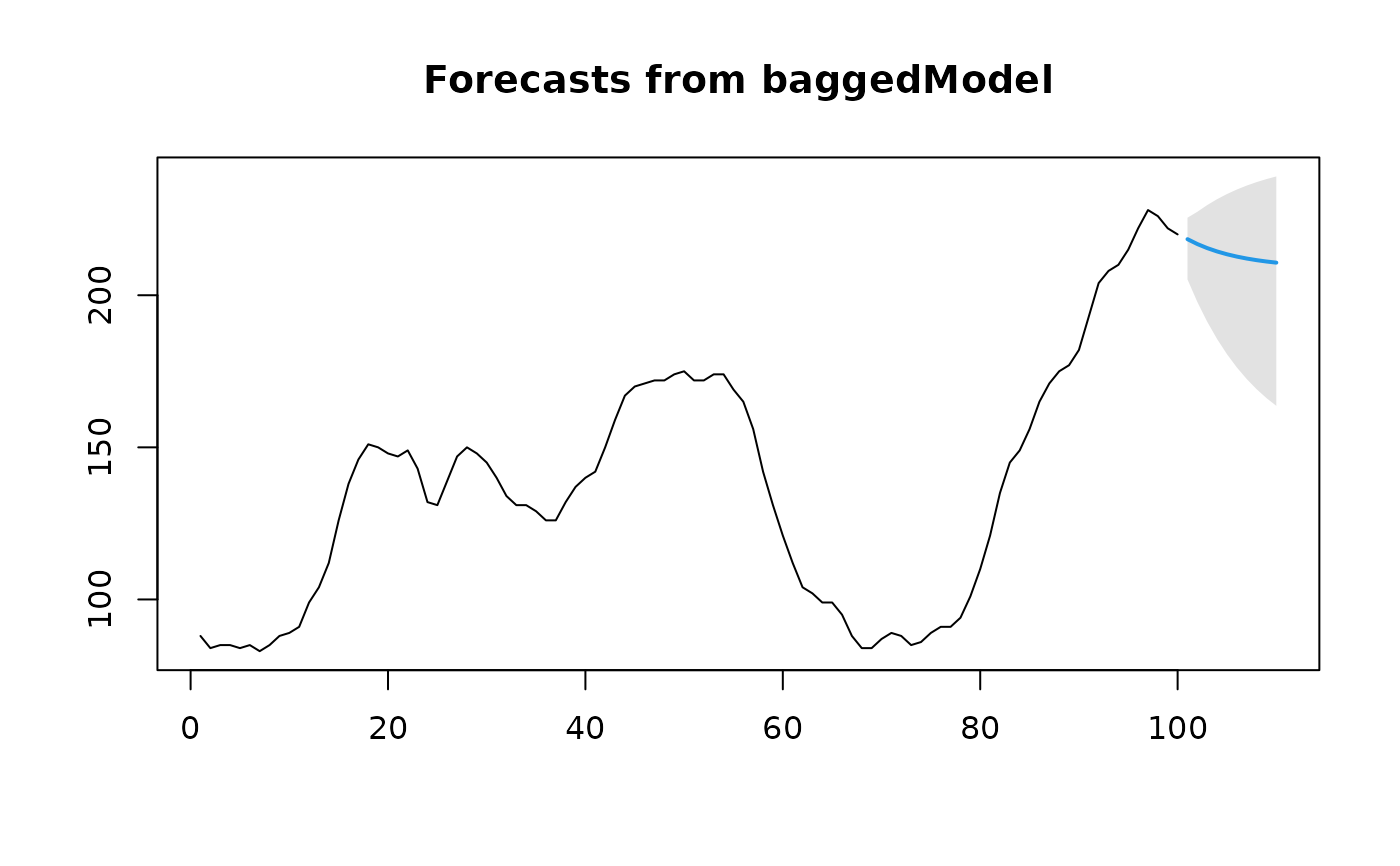Returns forecasts and other information for bagged models.

# S3 method for baggedModel
forecast(
object,
h = ifelse(frequency(object$y) > 1, 2 * frequency(object$y), 10),
...
)

## Arguments

object An object of class "baggedModel" resulting from a call to baggedModel. Number of periods for forecasting. Other arguments, passed on to the forecast function of the original method

## Value

An object of class "forecast".

The function summary is used to obtain and print a summary of the results, while the function plot produces a plot of the forecasts and prediction intervals.

An object of class "forecast" is a list containing at least the following elements:

model

A list containing information about the fitted model

method

The name of the forecasting method as a character string

mean

Point forecasts as a time series

lower

Lower limits for prediction intervals

upper

Upper limits for prediction intervals

level

The confidence values associated with the prediction intervals

x

The original time series (either object itself or the time series used to create the model stored as object).

xreg

The external regressors used in fitting (if given).

residuals

Residuals from the fitted model. That is x minus fitted values.

fitted

Fitted values (one-step forecasts)

## Details

Intervals are calculated as min and max values over the point forecasts from the models in the ensemble. I.e., the intervals are not prediction intervals, but give an indication of how different the forecasts within the ensemble are.

Bergmeir, C., R. J. Hyndman, and J. M. Benitez (2016). Bagging Exponential Smoothing Methods using STL Decomposition and Box-Cox Transformation. International Journal of Forecasting 32, 303-312.

baggedModel.

## Author

Christoph Bergmeir, Fotios Petropoulos

## Examples

fit <- baggedModel(WWWusage)
fcast <- forecast(fit)
plot(fcast)if (FALSE) {
fit2 <- baggedModel(WWWusage, fn="auto.arima")
fcast2 <- forecast(fit2)
plot(fcast2)
accuracy(fcast2)}